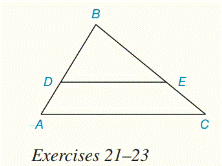Chapter 5.CR, Problem 21CRElementary Geometry For College St...

7th Edition
Alexander + 2 others
ISBN: 9781337614085

Solutions

Chapter
SectionElementary Geometry For College St...

7th Edition
Alexander + 2 others
ISBN: 9781337614085
Textbook Problem

For Review Exercises 21 to 23, D E ¯ | | A C ¯B D = 6 , B E = 8 , E C = 4 , A D = ?

To determine

To find:

Explanation

Given:

DE¯||AC¯

BD=6,BE=8,EC=4 and

Calculation:

Since, corresponding angles are equal, then

BDE=BAC(DE¯||AC¯)

BED=BCA(DE¯||AC¯)

Here, ΔDBE similar to ΔABC

Then,

Still sussing out bartleby?

Check out a sample textbook solution.

See a sample solution

The Solution to Your Study Problems

Bartleby provides explanations to thousands of textbook problems written by our experts, many with advanced degrees!

Get Started

Find more solutions based on key concepts